# Research Blog

Search
• danjhill95

## The Proofs are left in the Pudding for the Reader

I’m a big fan of mathematical proofs; one could argue that they are one of the few things that differentiate mathematics from other STEM subjects. It may not be the favourite topic of the average maths student, but there’s something special about being able to prove things in full generality. Also, creating your own proofs is a great exercise in logic, which is something I’d recommend for any maths students to at least try.

Here are some results that I've enjoyed finding proofs for in the past (which I’m leaving here for my own future reference). Some might be things that you were taught at school, and some others might surprise you:

1. Decimal expansion of one-seventh

First off, you may be aware that some fractions have a decimal expansion with infinitely repeating digits, such as 1/3 = 0.33333… One of the lesser known of these is 1/7, where its decimal has a repeating block of 6 digits “142857”. But how do we prove that this is true?

Let’s start by recognising that we can writeThen, we can do the same thing withFollowing this same approach, we see thatWhich, by dividing by the appropriate amount of 10’s, means thatApplying this recursively, we see that the 6-digit block “142857” just keeps on repeating infinitely.

2. A number is divisible by 3 if the sum of its digits is divisible by 3

This is a trick that many students learn to help with checking if a number is divisible by three but, other than being told this by our teachers, how do we know that this always works? Sure, we can check that it works for all numbers up to 200, say, but what if it stops working for all numbers larger than 1000? This is where a proof of full generality can really come into its own.

We first write the above statement in a bit more of a mathematical way. For some number A with N digits (so 134 would have N=3, 19928 would have N=5, etc.) we can write its decimal expansion aswhere each a, a, …, a[N-1] is the first, second, …, N’th digit of our number. So, for our previous examples,Then, our statement can be written as

A is divisible by 3 if and only if a + a + a + … + a[N-1] is divisible by 3.

To prove this, we recall that 10=9+1, and soWhich means that,Clearly, the last bracket is divisible by 3, since it is 9 times a collection of terms; hence, A is only divisible by 3 ifis divisible by 3. Playing the same trick, we find that A is only divisible by 3 ifis divisible by 3, and so on. Applying this N-1 times, we then arrive at the result.

3. The square root of 2 can't be written as a fraction of two whole numbers

Now this one, *slaps bonnet*, this is a classic. The fact that the square root of 2 cannot be written as a fraction of two whole numbers, which means that we call sqrt(2) irrational, is a staple of entry-level maths proofs in UK Universities. The thing that makes this problem so great is its accessibility. The proof itself only really uses properties of odd and even numbers.

To prove this, we’re going to use an idea known as proof by contradiction. What that means is, we are going to assume the statement is wrong and then follow the logic until we arrive at some contradiction. This would then mean that it is impossible for the statement to be wrong, and so it must be right.

Okay, so let’s assume that sqrt(2) can be written as a fraction of two whole numbers; that is, there exist some whole numbers p and q such thatNow, we can assume that p and q don’t have any common factors, since we could then simplify the fraction p/q by dividing through by this factor, i.e. if p=3*a, q=3*b for some whole numbers a and b, then we could write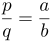The trick is to now square both sides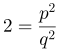And so, p^2 = 2*q^2. Therefore, p^2 is an even number, which also means that p is an even number. Then, we can writefor some whole number k, which means thatDividing by 2, we see thatwhich means that q^2, and thus q, is also even. But if q and p are both even, then they are both divisible by 2. This is a contradiction since p and q have no common factors. Hence, our assumption thatmust be wrong.

4. Pythagorean triples, quintuples, etc.

You may be aware of the identityfrom Pythagoras’s Theorem. Recently I saw the following result drifting around Math Twitter,Now, this kind of blew me away to start with, and it’s natural to ask `Is there a general version of this?’. In fact, there is, and so the statement I want to prove is the following:

For any whole number k>0, the equationhas one unique positive solution and one unique negative solution for a.

To prove this, I find it easier to first redefine the variable a; let’s introduce b=a+k such that the equation above becomesfor which we can rearrange into the following form: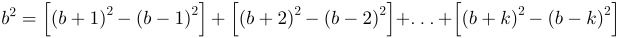We note thatand sowhich has two solutions: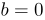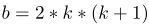Converting back to our variable a, we find thatare the two solutions. Hence, our equation has one unique positive solution and one unique negative solution. Since we’ve done this in full generality, we can now conclude thatand many more…

## 5. Checkerboard matrix negation is determinant-invariant

This final result is something I recently stumbled upon during some of my research, but it does have a bit more required knowledge regarding matrices. As such, I’m not going to go into the proof itself; instead, I’ll try to explain the result and then leave the proof as an exercise for any interested readers out there.

Firstly, it's important to know that a matrix is a collection of numbers in a row-column structure. For example,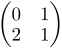is a 2x2 matrix (since there are 2 columns and 2 rows). For square matrices, where the number of rows and columns are the same, there is a measurement of the matrix known as the determinant that characterises its behaviour. Given a general 2x2 matrix A of the form,then the determinant is calculated asThe result I recently learned about is that, for any NxN matrix (where N is some positive whole number), negating every other element of the matrix, starting from the (row 1, column 2) element, leaves the determinant invariant. That is, given a matrix B of the formand let’s say that we define some new matrix C, of the form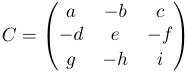Then det ( C ) = det ( B ). Note, this is true for any sized square matrix, which was something that astounded me.

I want to emphasise that, if you tried proving any of these results above as well as anything else, proofs are sometimes hard work. More than this, making a proof that is clear and easy to follow is even harder, and almost always requires multiple attempts. In fact, having noticed that the above result seemed to be true for the matrices I was working with, I managed to construct a 2-page proof by induction for the general case. Following this, I found an off-handed comment about this property in a paper where the author presented a two-line proof. So don’t be discouraged if you didn’t think up the most efficient proof for a particular result. The truth is, most of the proofs that you see are the results of multiple attempts, as well as multiple drafts to make them clear and concise.

• danjhill95

## A Tale of Family History, Calculators, and the Father of the Computer

One of the things that I love about family history are the small stories you learn along the way. Once you sift through all the farmhands and servants, you may occasionally come across a person who seems a bit more interesting. Suddenly, the focus is no longer about various lineages and families evolving across centuries. Instead, you find yourself looking through a window into the life of one individual. The questions are no longer just `Who is this person related to?’ or `Where did this person live?’, but also `What was it like to live in this period?’ and `Where did this person fit in society?’. Being able to ask these questions allows me to have a much deeper connection to the research, which can otherwise feel like an exercise in spreadsheets and convoluted filing systems. And I think that is pretty cool.

Now, throughout my historical research, these types of individuals are mostly based in the armed forces. This might just be because of the historically working-class nature of most of my family, or maybe because joining the armed forces is a life-altering decision that also happens to have a decent paper trail. Either way, it was a pleasant surprise to recently come across my great(x5) granduncle Joseph Clement, an engineer based in Southwark, London during the early 1800s. Though I had never heard of Joseph before, I soon discovered that he was involved in one of the most influential mathematical developments of the Victorian era. And, as I found out, it all starts with the machines that occupy most of our lives.

## The Machine Revolution

It is sometimes difficult to appreciate what life was like before machines. By 2025, the World Economic Forum predicts that half of all work tasks will be handled by machines, and nowadays the average person always has access to a digital calculator in their phones. But this is still a relatively recent development; the first all-electric calculators were released in the late 1950s, but portable calculators were not commercially available until the 1970s. I think it is interesting that the public understanding of calculating tools includes the abacus (originating before 2300 BCE) and electronic calculators, but there is a gaping chasm between these two technologies that seems to be often forgotten.

When I try to think of a mechanical calculator the first thing that comes to mind is an old-fashioned till, or cash register in the US, partly due to watching a significant amount of the British sitcom Open All Hours as a child. The first mechanical tills were simple examples of a calculator, since they usually only added numbers, and were first patented in 1883.

Along with the Arithmometer and Comptometer, which both entered production in the mid-to-late 1800s, mechanical tills helped to popularise the use of calculating machines in businesses. Over time, these machines expanded their capabilities, allowing for mathematical operations such as subtraction, multiplication, and division. But it all started with a simple adding machine.

## It’s all adding up now

Although calculating machines became commonplace in the late 1800s, mathematicians had been developing this technology for centuries prior. In 1642, 18-year-old Blaise Pascal was assisting his father in supervising the taxes of Rouen. To reduce his workload, Pascal designed a device known as the Pascaline that could add or subtract two numbers directly. This machine used a system of spoked wheels and was notable for being able to `carry the one’ across different digits.A drawing of Leibniz's `Stepped Reckoner' by Hermann J. Meyer

Gottfried Leibniz, of biscuit fame, continued this work by completing his `stepped reckoner’ in 1694. This device was supposed to add and subtract two numbers directly as well as being able to perform long multiplication and long division. Unfortunately, the machine proved to be unreliable and never progressed past the prototype stage. The operating mechanism, called the Leibniz wheel, continued to be used in calculating machines for the next 200 years, however.

During the 1700s, various new calculating machines were designed based off the work of Pascal and Leibniz. These machines often tried to reliably multiply and divide two numbers directly, and often failed. Part of the reason for this is that multiplication and division are hard. As numbers get larger, trying to multiply becomes a laborious process that we have developed tricks to speed up.

Imagine how you would solve 62 x 47, and then imagine how you would instruct a machine to solve the same problem. You might think to use long multiplication, box multiplication, or maybe some other method to find that 62 x 47 = 2914. In every case, however, you are probably using those multiplication tables that you had to memorise as a child, something that calculating machines do not have access to. We now have multiple ways to speed up this process for machines, including the use of a binary number system, but this was still a glaring problem for the calculating machines of the time.

Conveniently, an alternate way to multiply and divide numbers had been made possible in the 1600s, thanks to the discovery of logarithms. Logarithms can be defined for various base numbers, commonly 10, 2, or the exponential constant e, but they all share the same property that

log(a) + log(b) = log(a x b).

Then, you can calculate (a x b) by first adding the logarithms of a and b and then finding the number whose logarithm equals that addition. Let’s take the example of 62 x 47 again, and I will use the “common” logarithm (which just means base 10). Looking up the values for log(62) and log(47), I find that

log(62) = 1.79329, log(47) = 1.6721,

and so,

log(62 x 47) = 1.79329 + 1.6721 = 3.46449.

Similarly, I can look up what number has a logarithm of 3.46449, and I discover that

log(2914) = 3.46449.

So, if I can look up possible logarithm values, I can calculate (a x b) without ever actually doing multiplication, which could then be automated by a mechanical calculator of the time. Extensive tables of logarithms were produced by hand and proved exceedingly helpful for engineering and navigation, since calculations required a great degree of accuracy. However, these tables were known to contain errors, which could prove deadly for sailors. And so, in stepped Charles Babbage.

## Babbage makes the differenceCharles Babbage in 1860

Charles Babbage was a busy man. He reformed the British post system, invented the cowcatcher, and publicly crusaded against children rolling a hoop down the street. He was also a founding member of the Royal Astronomical Society, who had taken an interest in the problems regarding the accuracy of logarithm tables. Babbage argued that he could design a machine that would automate the production of these tables, and that this would ensure their accuracy.

His pitch was successful, and the British government provided him with £1700 (a little under £100,000 in today’s money) to begin work on his `difference engine’. The difference engine was ground-breaking in its complexity compared to other mechanical calculators; it had storage, where data was held for processing, and was designed to take up an entire room. Importantly, it would stamp its results into soft metal, which could be used for printing. This removed any possibility of errors in copying the results during typesetting, which Babbage saw as the main culprit for any errors in hand-written tables.

The engine used the principle of divided differences, hence its name, to compute values of a polynomial. To see this principle in action, let’s think about an example. Take a racing car driving down a straight racetrack; we don’t how fast the racing car is going, but we’ll assume that they are accelerating at a constant rate. The question is: if we know where the car is at certain times, can we predict where it will be in the future?

First, let's solve the problem by hand. By assuming that the car is accelerating at a constant rate, we can write down a formula for the distance s in terms of the time t, acceleration a, and initial speed u. The formula looks like this:

s = u x t + (1/2) x a x t^2,

where t^2 means t squared; this can be derived from calculus, though that isn’t important here. So far, we have a formula for the distance s, but we need to know how fast the car is accelerating and its initial speed. Let’s say we now measure the car’s distance at certain times, and we find that it has travelled 4 meters after one second and 12 meters after two seconds. Then, we could use these values to solve for u and a:

t = 1, 4 = u + (1/2) x a,

t = 2, 12 = 2 x u + 2 x a,

and we find that a=4 and u=2. This means that

s = 2 x t + 2 x t^2,

and so, the car will have travelled 24 meters after 3 seconds and 40 meters after 4 seconds. This is a reasonably straight-forward solution, but it required us to solve two simultaneous equations using some mathematical logic. So how do you get a machine to solve this problem?

To solve this problem, we can use a technique known as finite difference. We begin by defining the first difference d1( t ) = s( t + 1 ) - s( t ), which is effectively the change in distance travelled in the next second, and the second difference d2( t ) = d1( t + 1 ) - d1( t ), which is effectively the change in average speed in the next second. If we return to the general formula for the distance s in terms of time t, acceleration a, and initial speed u,

s(t) = u x t + (1/2) x a x t^2,

we note that,

d1(t) = u + a x t + (1/2) x a, d2(t) = a.

The important thing here is that d2(t) is constant, i.e. it doesn’t depend on the time t. This is true for all quadratic polynomials (that is, where t^2 is the highest power of t). In fact, for any polynomial where t^m is the highest power, the m-th difference is constant; this is the basis behind Babbage’s design for the difference machine.

We can write down a table for the distances and first and second differences for our earlier hypothetical measurements,

t s(t) d1(t) d2(t)

------------------------------------------------

0 0 4 4

1 4 8

2 12

Then, since the fourth column is constant, we can start to fill in the missing values recursively. This means that, by adding each cell to its right neighbour we can compute the value of the cell below it. So, d1(2) = d1(1) + d2(1) = 8 + 4 = 12, and so on:

t s(t) d1(t) d2(t)

-------------------------------------------------------------------------------------------

0 0 4 4

1 4 8 4

2 12 (8+4) = 12 4

3 (12+12) = 12 (12+4) = 16 4

4 (24+16) = 40 (16+4) = 20 4

5 (40+20) = 60 (20+4) = 24 4

which we can check with our previous answer that we found by hand. Hence, a machine with (m+2) columns can accurately tabulate the values of any polynomial up to a power of m, given that you have (m+1) initial values. Many more complicated functions (such as logarithms and trigonometric functions) can be approximated by polynomials, and so Babbage’s design appeared to be the solution to the error-filled logarithm tables that plagued British sailors. However, things are never that quite that simple.

## You just can’t get the staff these days!

Much like with the mechanical calculators of the 1600s, the construction of the difference engine was hampered by the engineering capabilities of the time. Babbage was very serious about the project: he built a dust-proof environment to test the machine, set up a fire-proof workshop, and hired master machinist Joseph Clement (remember him from earlier?).

Westmorland-born, Clement was famous in London at the time for his high-precision tools, including lathes and planers. By 1832, Babbage and Clement produced a working model of one-seventh of the full engine, which could compute second-order differences with up to six-digit numbers. This portion of the engine consisted of about 2,000 parts and can still be seen at the Science Museum in London.A Photo of the Difference Engine constructed by the Science Museum. Source: User:geni, CC BY-SA 4.0, via Wikimedia Commons

However, in 1833 Babbage and Clement had a falling-out – supposedly Babbage thought that Clement was using the projects funds to improve his own workshop, while Clement refused to continue his work unless Babbage paid for the tools required to build the engines parts. Whatever the reason, work on the engine was suspended and the project was abandoned in 1842; in this time, £17,000 (just under £1 million in today’s money) of governmental funds had been invested into the project, and a working engine was still no closer. It is fair to say that, in the eyes of the British Government, Babbage’s difference engine was a complete and utter failure.

## Rise of the Machines

But of course, Babbage’s story does not end there. During the difference engine project, Babbage began work on a more general design, known as the analytical engine. This machine would be provided with initial data and programs via punched cards and could then perform any number of arithmetic tasks. The logical structure of the analytical engine is essentially the same as modern-day computers, but the significance of this work was lost on many scientists at the time.

One person who saw the potential in this machine was Ada Lovelace, a friend of Babbage’s who had been inspired by the difference engine prototype built by Clement. In 1843, Lovelace translated an article by Italian mathematician Luigi Menabrea on the analytical engine and, with it, attached a set of her own notes. These notes almost doubled the article itself and included a detailed algorithm for the engine to calculate Bernoulli numbers. This algorithm is often seen as the first computer program, and Lovelace as the first computer programmer.

While Babbage focused on the capabilities of machines to calculate functions and crunch numbers, Lovelace saw the potential far beyond this. She observed that machines may be able to compute things other than numbers, if those things satisfied mathematical rules. In an age where machines determine large portions of everyday society, it is hard to disagree with her.

And so, that is where my research ends for now. I began with the life of Joseph Clement, a small cog in a machine that spent part of his life building small cogs for a machine. But in that life, I saw the efforts of multiple scientists (such as Pascal, Leibniz, and the engineers of the early 1800s) converge in one of the most ambitious feats of engineering of its time. And though the project was a failure, its influence, thanks to Charles Babbage and Ada Lovelace, can still be seen in computers to this day. Hundreds of years of mathematics and engineering distilled into one story. And, as I said, I think that is pretty cool.

• danjhill95

## And so it begins...

So, I've finally done it. It only took the entirety of my PhD in order for me to create my own website. Since this is the first of (hopefully!) many updates to this blog, let me set a baseline of where I am at at the moment:

• I submitted my PhD thesis on 31st March 2021 and my viva is scheduled for 10th June 2021 (Hooray!)

• My 74-page paper with David Lloyd and Matt Turner on localised radial patterns in the ferrofluid experiment has been accepted by the Journal of Nonlinear Science (Hooray!)

• I've applied for some postdoctoral jobs (Hooray?)

• I've been rejected by some, but not yet all, of the jobs I've applied for (hooray...)

• David Lloyd and I have been putting together a paper on the existence of localised cellular patterns in the Swift-Hohenberg equation (Hooray!)

• I'm currently also putting together a paper on localised radial patterns in a model for desert vegetation, to be part of an IMA special issue (Hooray!)

• I also built my personal website (Hooray!)

Thus, there's been much progress but there's still a lot of work to get done, it seems...

Some things to look forward to this month:

• I'm giving an online talk to the Leeds Applied Nonlinear Dynamics (LAND) Seminars on the 11th May. It's my first talk where I'm including some of the work on cellular patterns, so that should be fun!

• I'm attending the SIAM Conference on Applications of Dynamical Systems (DS21) at the end of May, where I'm giving a contributed talk on the ferrofluid problem.

And many more exciting things in future months. So for now, all the best!

Dan